### Keyword MAGNETIZABILITY

This keyword activates the calculation of closed-shell magnetizabilities.
Options:
 GTENSOR Calculation of the rotational g-tensor.
Description:
The magnetizability tensor can be calculated either with AUXIS or BASIS GIAO formalism . One or the other formalism is activated by the AUXIS or BASIS options of the VXCTYPE keyword. The default output prints the magnetizability tensor in atomic units and the isotropic and anisotropic magnetizability in both atomic units and SI units [JT]. The isotropic magnetizability is calculated as: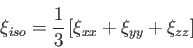(31)

The corresponding anisotropic magnetizability is calculated in the principal axis system of the magnetizability tensor as:(32)

To print the diamagnetic and paramagnetic magnetizability tensors the input line PRINT MAG must be used (see 4.12.2). The magnetizability along a BOMD trajectory is calculated in two steps as discussed in Section (4.7.3). A BOMD magnetizability calculation is activated by the option MAGNETIZABILITY of the SIMULATION keyword (see 4.7.3). In the following example, the magnetizability of ethane along a BOMD trajectory is calculated:

 TITLE ETHANE - MAGNETIZABILITY MD SIMULATION
#
BASIS (AUG-CC-PVDZ)
VXCTYPE AUXIS PW91
TRAJECTORY RESTART PART=10000-110000
SIMULATE CALCULATE MAG INT=25
#
GEOMETRY Z-MATRIX ANGSTROM
C                                   6    12.011000
H   1  R1                           1     1.007940
H   1  R2   2  A1            RAD    1     1.007940
H   1  R3   2  A2   3  D1    RAD    1     1.007940
C   1  R4   2  A3   3  D2    RAD    6    12.011000
H   5  R5   1  A4   2  D3    RAD    1     1.007940
H   5  R6   1  A5   2  D4    RAD    1     1.007940
H   5  R7   1  A6   2  D5    RAD    1     1.007940
#
VARIABLES
R1          1.10761992
R2          1.10778711
R3          1.10781183
R4          1.51627843
R5          1.10773895
R6          1.10766207
R7          1.10782491
A1          107.574370
A2          107.515075
A3          111.387913
A4          111.403485
A5          111.398744
A6          111.311745
D1         -115.468944
D2          122.337112
D3           59.891940
D4          180.000000
D5          -60.021828
#
END


The GTENSOR option of the magnetizability keyword activates the calculation of the rotational g-tensor , a dimensionless quantity, whose calculation requires the magnetizability tensor. In the default output, aside from the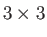rotational g-tensor calculated in the principal axis system of the tensor of inertia, its isotropic and anisotropic values are also printed: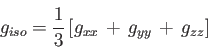(33)

and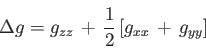(34)

The diagonal elements of the g-tensor are ordered according to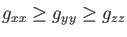. Together with the magnetizability the rotational g-tensor can also be calculated along a BOMD trajectory. It is activated by adding the following line to the above example of the magnetizability MD simulation:

 MAGNETIZABILITY GTENSOR